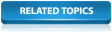# Channels

Channel objects can be used to model man made channels or a simplistic representation of a river channel.

(For detailed modelling of river channels, a River Reach object should be used. Channel objects can be automatically converted to river reach objects; see Converting Channels to River Reaches for details.)

The cross section of a channel is defined as a Channel Shape object. You can create new channel shapes or re-use an existing channel shape by selecting it from the Shape ID list in the Channel Properties Window.

## Hydraulic Model Characteristics

• The channel is considered to be prismatic. That is, the cross-section is the same from the upstream end to the downstream end.
• Each section of the channel is considered to be trapezoidal for the purposes of width, area, and wetted perimeter calculations.
• The conveyance in the channel is the sum of the conveyances in each panel (see Chow, VT (1959)).
• InfoWorks ICM uses the equivalent grain roughness concept (HR Wallingford and D.I.H. Barr (1994)) to determine the composite roughness of each panel. The same concept is used in conduits.
• If the left bank depth is not the same as the right bank, the channel is assumed to have a smooth vertical bank at the lower of the two banks.
• The capacity of the channel is defined for the highest depth defined in the section.
• Conveyance continues to increase above the maximum cross-section height. Conveyance is interpolated by the engine using a 6 point table of conveyance values between the highest section point and a fixed depth (3m) above the highest section point. If depth exceeds the tabulated height, conveyance is calculated by extrapolation from the last two table entries. Warning messages will be displayed in the log results if depth exceeds section height or maximum tabulated height.
• Wetted perimeter does not increase above the highest section point.
• Width is constant above the highest section point.
• We define the height of the channel as the largest depth in the section.
• We define the width of the channel as the difference between the last and first X coordinates.

### Erosion / Deposition model

The erosion/deposition model evaluates friction factors for the sediment bed, lower third of the conduit, and upper two-thirds of the conduit (to match the way we define roughness in conduits).  To minimise changes to this model, we calculate composite roughness values for the lower 1/3 and upper 2/3 sections of each channel inside the simulation engine.

How we do this:

1. Calculate conveyance at 1/3 channel depth.
2. Use the Manning equation to determine an assumed constant n value up to 1/3 channel depth.
3. Calculate conveyance at channel depth.
4. Use the Manning equation to determine an assumed constant n value up to channel depth.
5. Use the equivalent roughness concept to determine the n roughness value for the upper 2/3 of the channel.

We use the Manning equation because we can use a simple direct method to determine n.

### Base flow

The default base flow depth for both a conduit and a channel is the sediment depth + 5% (Base Flow Factor) of the conduit height minus the sediment depth. This is increased to 10% for conduits of slope greater than 0.01 (Slope Where Base Flow Is Doubled). This is subject to a minimum base flow depth of 0.02m (Minimum Base Flow Depth).  You can change these defaults for a particular network in the Simulation Parameters.

For a channel, defining a base flow of 5% of the channel depth may have an excessive impact on hydraulic results, especially if your cross-section definition includes the flood plain.  So you can set a user-defined value of base flow depth in each channel.

Normally, a depth of a few centimetres should suffice. If your simulation results contain depth spikes, increasing the base flow depth may help.

If you set the user defined base flow depth to zero then InfoWorks ICM will calculate a base flow depth from the simulation parameters (see above).  A non-zero base flow depth will be reset to the minimum base flow depth, if it is lower.